# 曦行夜落 的博客# 曦行夜落 的博客

### 浅谈二分的边界问题

posted on 2018-07-18 23:07:47 | under 文章 |

## 前言：二分简介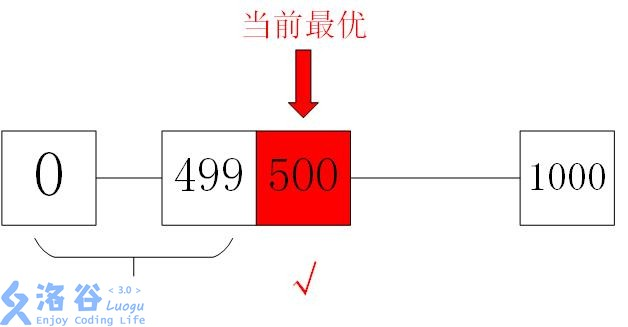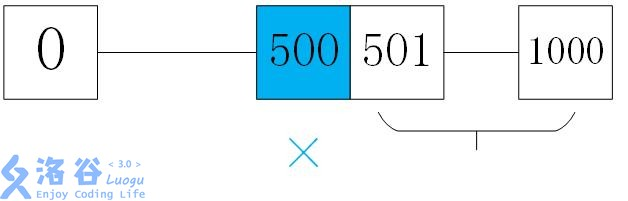## Part1：二分的中心思想

1. l和r代表的“成本值”均可行，且有一个ans变量记录当前的最优
2. l和r代表的“成本值”均可行，最后的答案是l或r
3. l代表的“成本值”可行，r不可行，最后的答案是l

## Part2：写法一——记录答案法】

while (l<=r)
{
int mid=(l+r)/2;
if (check(mid))
{
ans=mid;
r=mid-1;
}
else l=mid+1;
}
printf("%d",ans);

check(mid)是一个bool函数，返回mid是否可行，下面就是分情况讨论

1. mid可行，此时记录ans=mid，也就是mid是目前最优解，然后缩小查找范围：r=mid-1，寻找有没有更小的解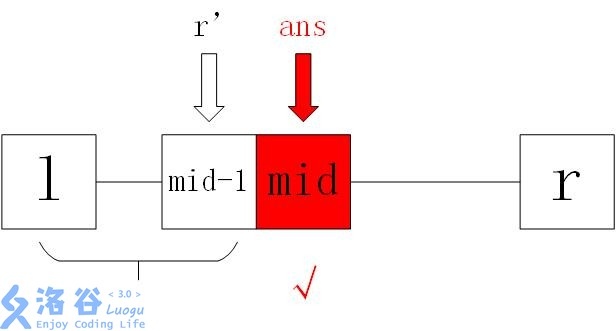2. mid不可行，此时令l=mid+1，找更大的解来满足条件也就是说，当mid可行，就记录mid，然后找有没有更优的，否则就退而求其次，牺牲数据的优秀度来满足条件

1. 可以，记下他，然后对比他弱的人进行测试（ans=mid r=mid-1）
2. 不行，对比他强的人进行上述操作（l=mid+1）

## Part3：写法二——不记录法

while (l<r)
{
int mid=(l+r)/2;
if (check(mid)) r=mid
else l=mid+1;
}
printf("%d",l); //r也可以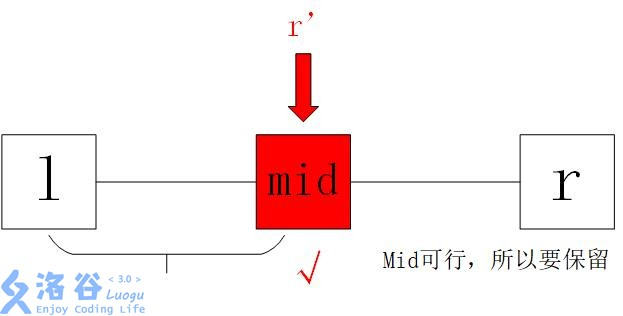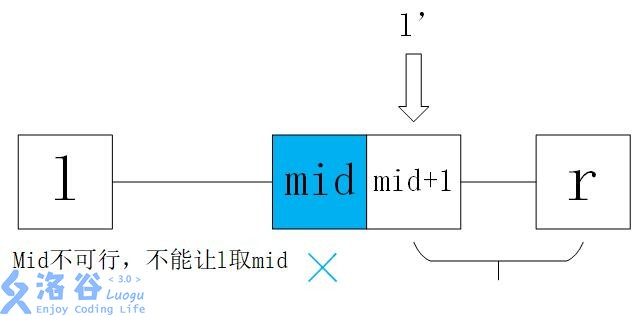**打个比方：一群选手按实力顺序排好，然后我们要找出能够AK IOI的实力最差的选手，那么我们选实力居中的那个，测试他能不能AK IOI：

1. 可以（check(mid)=true）。那么实力比他更强的选手可以回去了，因为他们一定能AK IOI，而且实力比刚刚测试的那位要高。但是那个测试过的就可以“暂时安全”，因为他是目前最优解（能AK且实力最差），这一个操作就是r=mid
2. 不能（check(mid)=false）。那么他以及实力比他弱的全部淘汰，这个操作是l=mid+1
然后用新的区间不断重复即可

1. 可以（check(mid)=false）。此时他以及比他更强的都不能符合要求（我们要找不能AK的，不是AKer），所以r=mid-1
2. 不能（check(mid)=true）。此时比他弱的人全部淘汰（比他弱的更不能，而且实力比他弱），这个操作是l=mid
之后就是对新的区间进行一样的操作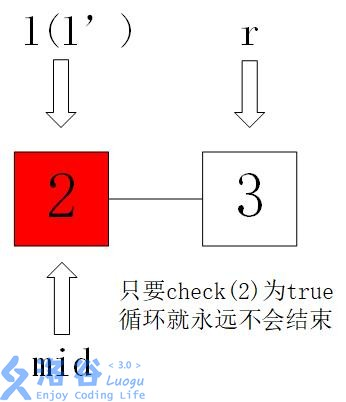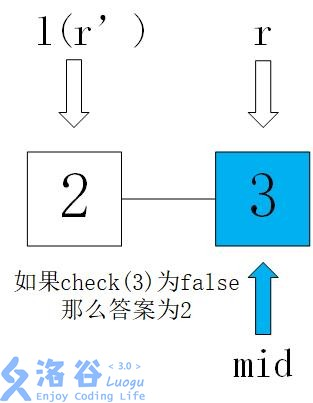## Part4：二分查找

1. 查找值为key的元素下标，不存在返回-1

int l=1,r=n;
while (l<=r)
{
int mid=(l+r)/2;
if (a[mid]==key) return mid;
else if (a[mid]>key) r=mid-1;
else l=mid+1;
}
return -1;

1. 查找大于等于/大于key的第一个元素
int l=1,r=n;
while (l<r)
{
int mid=(l+r)/2;
if (a[mid]>=key) r=mid; //如果要求大于，可以将=去掉
else l=mid+1;
}
return l;

。那么，我们按照之前讲到的，如果一个人可以AK（a[mid]>=key），那么比这个人强的人必然可以AK（对于所有i>mid，a[i]>=key），而且不会更优，所以不要，就让r=mid

1. 查找小于等于/小于key的最后一个元素
int l=1,r=n;
while (l<r)
{
int mid=(l+r)/2;
if (a[mid]<=key) l=mid; //如果求小于，可以去掉=
else r=mid-1;
}
return l;

## Part5：二分答案例题——丢瓶盖（洛谷P1316）

• 问题——不能AK的最强选手（可以选出B个瓶盖的最大距离）
• 不能AK——可以选出B个瓶盖符合条件
• 水平——最大距离
• 如果居中的那个人不能AK（当前的距离限制可以选出B个瓶盖满足条件），那么令l=mid，留下他和水平更高的（往大了找）
• 如果居中的那个人可以AK（当前的距离限制不允许选出B个瓶盖满足条件），那么令r=mid-1，留下水平更低的

#define maxn 100000+500
#include<cstdio>
#include<algorithm>
using namespace std;
int n,k;
int a[maxn];

int check(int m)
{
int tmp=a+m,cnt=1;
for (int i=2;i<=n;++i)
if (a[i]>=tmp)
{
cnt++;
tmp=a[i]+m;
}
return cnt>=k;
}

int main()
{
scanf("%d%d",&n,&k);
int l=1,r=1000000000;
for (int i=1;i<=n;++i)
scanf("%d",&a[i]);
sort(a+1,a+1+n);
//  for (int i=1;i<=n;++i) printf("%d ",a[i]); printf("\n");

int ans=0;
while (l<r)
{
int mid=(l+r+1)/2;
if (check(mid)) l=mid;
else r=mid-1;
}
printf("%d",l);
return 0;
}